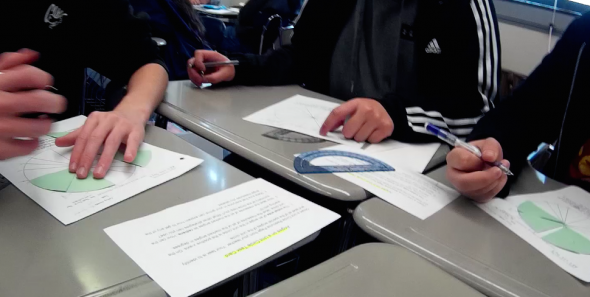# Algebra 2 + Precalculus## Algebra 2 + Precalculus

Landing page for parents to learn about Algebra 2 + Precalculus

In this compression course, instructional time should focus on three critical areas:

1. expand understandings of functions and synthesize and generalize function properties to transform a variety of functions;
2. extend the domain of trigonometric functions using the unit circle and model periodic phenomena with trigonometric functions; and
3. develop understanding of essential topics from Precalculus to prepare students for AP Calculus.